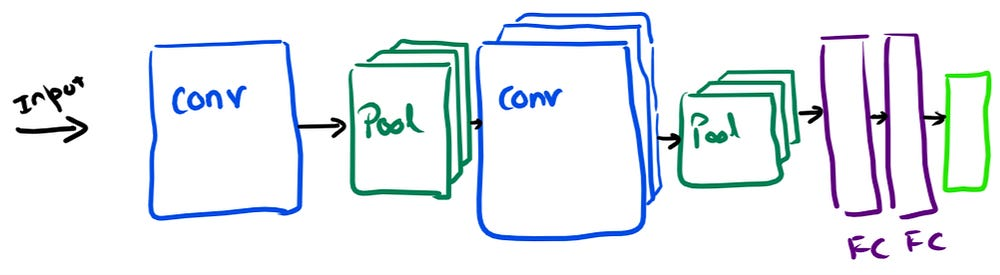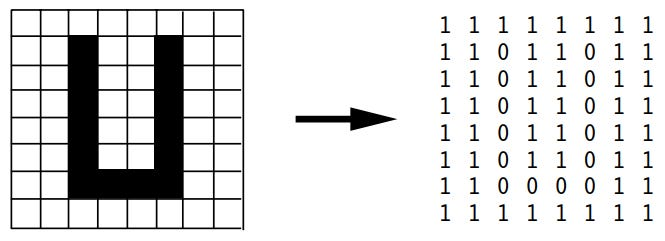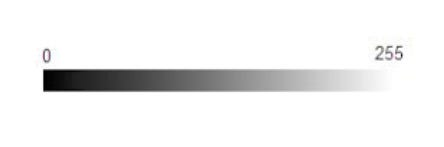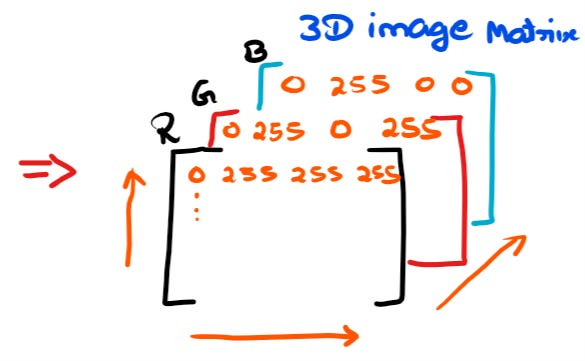# Beginner to Advance: Convolutional Neural Networks Made Easy (Part -1)

Source: Deep Learning on Medium

This blog is a part of ongoing Beginner to Advance series for anyone starting from scratch on deep learning (CNN)!!

Convolutional neural networks (CNN) are universally used in the field of computer vision applications, they are also known as Convnets. CNN models can do image classification with more accuracy than humans… yup !!

#### Architecture:

This is to give you a birds-eye view of a CNN model. In a CNN model, an input image goes through a number of convolutional & pooling operations, followed by fully connected layers. We will delve in the nitty-gritty of each component later.Convolutional Neural Network Model: Figure 1

Convolution is a mathematical operation on two functions (f and g) to produce a third function that expresses how the shape of one is modified by the other… dont worry about the tough lanugauge it is what wikipedia does to simple concepts:)

#### Elements of CNN Model:

1. Input: Input to CNN is generally image & images are just numbers.

Black & White Image- They are called logical images and have two colors, black (represented as 0), white(represented as 1). Black & white image can be represented as a 2D matrix of numbers.Black & White Image (MxN) Figure 2

Greyscale Images- They have the intensity of grey (shades of grey ) represented by numbers between [0,255] at every pixel. Greyscale can also be represented as a 2D matrix of MxN. Each element in the image will have a value between 0 to 255 (0 for black, 255 for white)Grayscale image : Values between 0 to 255, Figure 3

Color Image- When you create a matrix for color images or RGB images, it contain three planes corresponding to each of one color Red, Green, Blue (RGB). A color image can be represented by a 3D matrix (3D tensor, 4D in case of batch size/multiple images) having height, width, color depth. Each element in the matrix is one Byte (8 bits) & have value between 0 to 255.RGB image equivalent matrix : Image Source, Figure 5

2. Convolution Layer: They are the workhorses in CNN model, real magic happens here. Convolution is an operation that merges two set of information. Here convolution is applied on the input matrix using a convolution filter to produce a feature map.

Example: On the left hand side you can see the input to the convolution layer (assuming a small image 6x6x3), here Channel(C) = 3 as it is a RGB image. Filter or kernel is having a dimension of 3x3x3 i.e 27 numbers. Channel in filter will be equal to channel in Input… remember this !!

We do matrix wise multiplication at every location and sum the output. It is a volume multiplication(As per the example 27×27 elements) This sum goes in the output image or feature map. Filter/Kernel is slided all over the image to fill the output feature map.

Stride: Stride specifies how much we move the filter/kernel over the input image. If stride is equal to 1 then filter slides one pixel/unit at a time.

Receptive field: Area where the convolution operation takes place is called as receptive field.

We use multiple filters and can stack the feature maps in the output. In the example you see three filters being used and resultant feature map is having three channels (4x4x3).

3. Non-linearity/Relu layer: Non- linearity isn’t its own distinct layer however is part of convolution layer. we pass the output of convolution operation through Relu activation function (Please check my blog on activation functions for details). Values in the final feature map are not actually the sums, but the Relu operation applied on them.

4. Padding: Stride specifies how much filter/kernel is moved over the input image, bigger strides result in less overlap between the receptive fields but it results in the feature map getting smaller and smaller after every convolution operation, which is not appreciable in CNN. Hence to keep the input image dimensions same as feature map we add padding in the input. Padding in CNN preserves the feature map dimension. We pad the input with zeros or some value around the edges.

5. Pooling Layer:

In a deep CNN model like VGGnet (16 layers) there are 138357544 trainer parameters. A pooling layer downsample the height and width of feature map keeping the depth intact. Pooling layer reduces the number of learned parameters which reduces overfitting and reduce training time. There are many type of pooling like max pooling, sum pooling, sum pooling however max pooling is most common.

Max pooling: It slides over the input (Output of convolution layer i.e. feature map ) & at every location maximum value is selected.

Here max pooling have halved the input feature map dimension (& reduced the total number of parameters to 1/4th of the input) while preserving the important information gained during convolution operation. remember depth will not change, channels remain same !!

In CNN architectures, pooling is typically performed with 2×2 windows, stride 2 and no padding. While convolution is done with 3×3 windows, stride 1 and with padding.

6. Fully connected layers:

Fully connected layer takes an 1D input, so the 3D output of the final pooling layer is flatten (flatten is an operation) and fed into fully connected layer. In case of multiclass classification activation function can be softmax here.

Summary: